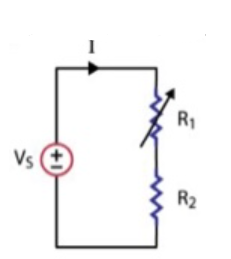# Problem: Given: The circuit below, R2 = 20 kOhms, VS = 12V, and R1 starts at 100 kOhms and is turned down to 20 kOhms. Find: a) the power absorbed in R2 when R1 = 100 kOhms, and b) the power absorbed in R2 when R1 = 20 kOhms.

###### FREE Expert Solution

We are dealing with power in circuits.

Power in circuits:

$\overline{){\mathbf{P}}{\mathbf{=}}{\mathbf{V}}{\mathbf{i}}{\mathbf{=}}{{\mathbf{i}}}^{{\mathbf{2}}}{\mathbf{R}}{\mathbf{=}}\frac{{\mathbf{V}}^{\mathbf{2}}}{\mathbf{R}}}$

We also have resistors in series.

86% (373 ratings)###### Problem Details

Given: The circuit below, R2 = 20 kOhms, VS = 12V, and R1 starts at 100 kOhms and is turned down to 20 kOhms. Find: a) the power absorbed in R2 when R1 = 100 kOhms, and b) the power absorbed in R2 when R1 = 20 kOhms.